# CorrelationMatrix¶

class CorrelationMatrix(*args)

Correlation Matrix.

Available constructors:

CorrelationMatrix(dim)

CorrelationMatrix(dim, values)

Parameters:
diminteger

The dimension of the correlation matrix (square matrix with dim rows and dim columns).

valuessequence of float

Collection of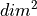scalar values to put in the correlation matrix, filled by rows. When not specified, the correlation matrix is initialized to the identity matrix.

Notes

In the first usage, the correlation matrix is the identity matrix.

In the second usage, the correlation matrix contains the specified values, filled by rows.

Warning

No check is made on the values, in particular the diagonal elements are not forced to be equal to 1 and the positiveness of the matrix is not checked.

Methods

 Check if the internal representation is really symmetric. clean(threshold) Set elements smaller than a threshold to zero. computeCholesky([keepIntact]) Compute the Cholesky factor. computeDeterminant([keepIntact]) Compute the determinant. computeEV([keepIntact]) Compute the eigenvalues decomposition (EVD). computeEigenValues([keepIntact]) Compute eigenvalues. computeGram([transpose]) Compute the associated Gram matrix. Compute the Hadamard product matrix. Compute the largest eigenvalue module. computeLogAbsoluteDeterminant([keepIntact]) Compute the logarithm of the absolute value of the determinant. computeQR([fullQR, keepIntact]) Compute the QR factorization. Compute the regularized Cholesky factor. computeSVD([fullSVD, keepIntact]) Compute the singular values decomposition (SVD). computeSingularValues([keepIntact]) Compute the singular values. Compute the sum of the matrix elements. Compute the trace of the matrix. Accessor to the object's name. Get the k-th diagonal of the matrix. Get the k-th diagonal of the matrix. Accessor to the dimension (the number of rows). Accessor to the object's id. Accessor to the underlying implementation. Accessor to the object's name. Accessor to the number of columns. Accessor to the number of rows. Test whether the matrix is diagonal or not. Tell if the matrix is empty. Test whether the matrix is positive definite or not. reshape(newRowDim, newColDim) Reshape the matrix. reshapeInPlace(newRowDim, newColDim) Reshape the matrix, in place. setDiagonal(*args) Set the k-th diagonal of the matrix. setName(name) Accessor to the object's name. solveLinearSystem(*args) Solve a square linear system whose the present matrix is the operator. Solve a rectangular linear system whose the present matrix is the operator. Square the Matrix, ie each element of the matrix is squared. Transpose the matrix.
__init__(*args)
checkSymmetry()

Check if the internal representation is really symmetric.

clean(threshold)

Set elements smaller than a threshold to zero.

Parameters:
thresholdfloat

Threshold for zeroing elements.

Returns:
cleaned_matrixMatrix

Input matrix with elements smaller than the threshold set to zero.

computeCholesky(keepIntact=True)

Compute the Cholesky factor.

The Cholesky factor of a covariance (real symmetric positive definite) matrix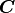is the lower triangular matrix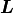such that: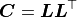Parameters:
keep_intactbool, optional

A flag telling whether the present matrix can be overwritten or not. Default is True and leaves the present matrix unchanged.

Returns:
cholesky_factorTriangularMatrix

The left (lower) Cholesky factor.

Notes

This uses LAPACK’s DPOTRF.

computeDeterminant(keepIntact=True)

Compute the determinant.

Parameters:
keep_intactbool, optional

A flag telling whether the present matrix can be overwritten or not. Default is True and leaves the present matrix unchanged.

Returns:
determinantfloat

The square matrix determinant.

Examples

>>> import openturns as ot
>>> A = ot.SquareMatrix([[1.0, 2.0], [3.0, 4.0]])
>>> A.computeDeterminant()
-2.0

computeEV(keepIntact=True)

Compute the eigenvalues decomposition (EVD).

The eigenvalues decomposition of a square matrix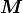with size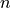reads: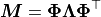where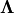is an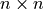diagonal matrix and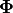is anorthogonal matrix.

Parameters:
keep_intactbool, optional

A flag telling whether the present matrix can be overwritten or not. Default is True and leaves the present matrix unchanged.

Returns:
eigenvaluesPoint

The vector of eigenvalues with sizethat form the diagonal of thematrixof the EVD.

PhiSquareComplexMatrix

The left matrix of the EVD.

Notes

This uses LAPACK’S DSYEV.

Examples

>>> import openturns as ot
>>> import numpy as np
>>> M = ot.SymmetricMatrix([[1.0, 2.0], [2.0, -4.0]])
>>> eigen_values, Phi = M.computeEV()
>>> Lambda = ot.SquareMatrix(M.getDimension())
>>> for i in range(eigen_values.getSize()):
...     Lambda[i, i] = eigen_values[i]
>>> np.testing.assert_array_almost_equal(Phi * Lambda * Phi.transpose(), M)

computeEigenValues(keepIntact=True)

Compute eigenvalues.

Parameters:
keep_intactbool, optional

A flag telling whether the present matrix can be overwritten or not. Default is True and leaves the present matrix unchanged.

Returns:
eigenvaluesPoint

Eigenvalues.

Examples

>>> import openturns as ot
>>> M = ot.SymmetricMatrix([[1.0, 2.0], [2.0, -4.0]])
>>> print(M.computeEigenValues())
[-4.70156,1.70156]

computeGram(transpose=True)

Compute the associated Gram matrix.

Parameters:
transposedbool

Tells if matrix is to be transposed or not. Default value is True

Returns:
MMTMatrix

The Gram matrix.

Notes

When transposed is set to True, the method computes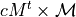. Otherwise it computes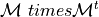Examples

>>> import openturns as ot
>>> M = ot.Matrix([[1.0, 2.0], [3.0, 4.0], [5.0, 6.0]])
>>> MtM = M.computeGram()
>>> print(MtM)
[[ 35 44 ]
[ 44 56 ]]
>>> MMt = M.computeGram(False)
>>> print(MMt)
[[  5 11 17 ]
[ 11 25 39 ]
[ 17 39 61 ]]


Parameters:
MatMatrix

The right hand matrix

Returns:
CMatrix

Notes

The matrix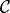resulting from the Hadamard product of the matrices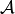and :cB is as follows: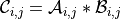Examples

>>> import openturns as ot
>>> A = ot.Matrix([[1.0, 2.0], [3.0, 4.0]])
>>> B = ot.Matrix([[1.0, 2.0], [3.0, 4.0]])
>>> print(C)
[[  1  4 ]
[  9 16 ]]
[[  1  4 ]
[  9 16 ]]

computeLargestEigenValueModule(*args)

Compute the largest eigenvalue module.

Parameters:
maximumIterationsint, optional

The maximum number of power iterations to perform to get the approximation. Default is given by the ‘Matrix-LargestEigenValueIterations’ key in the ResourceMap.

epsilonfloat, optional

The target relative error. Default is given by the ‘Matrix-LargestEigenValueRelativeError’ key in the ResourceMap.

Returns:
largestEigenvalueModulefloat

The largest eigenvalue module.

Examples

>>> import openturns as ot
>>> M = ot.SymmetricMatrix([[1.0, 3.0], [3.0, 4.0]])
>>> M.computeLargestEigenValueModule()
5.8541...

computeLogAbsoluteDeterminant(keepIntact=True)

Compute the logarithm of the absolute value of the determinant.

Parameters:
keep_intactbool, optional

A flag telling whether the present matrix can be overwritten or not. Default is True and leaves the present matrix unchanged.

Returns:
determinantfloat

The logarithm of the absolute value of the square matrix determinant.

signfloat

The sign of the determinant.

Examples

>>> import openturns as ot
>>> A = ot.SquareMatrix([[1.0, 2.0], [3.0, 4.0]])
>>> A.computeLogAbsoluteDeterminant()
[0.693147..., -1.0]

computeQR(fullQR=False, keepIntact=True)

Compute the QR factorization. By default, it is the economic decomposition which is computed.

The economic QR factorization of a rectangular matrixwith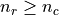(more rows than columns) is defined as follows: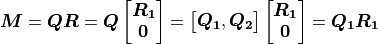where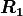is an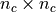upper triangular matrix,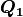is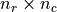,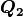is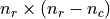, andandboth have orthogonal columns.

Parameters:
full_qrbool, optional

A flag telling whether Q, R or Q1, R1 are returned. Default is False and returns Q1, R1.

keep_intactbool, optional

A flag telling whether the present matrix is preserved or not in the computation of the decomposition. Default is True and leaves the present matrix unchanged.

Returns:
Q1Matrix

The orthogonal matrix of the economic QR factorization.

R1TriangularMatrix

The right (upper) triangular matrix of the economic QR factorization.

QMatrix

The orthogonal matrix of the full QR factorization.

RTriangularMatrix

The right (upper) triangular matrix of the full QR factorization.

Notes

The economic QR factorization is often used for solving overdetermined linear systems (where the operatorhas) in the least-square sense because it implies solving a (simple) triangular system: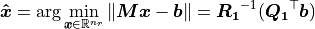This uses LAPACK’s DGEQRF and DORGQR.

Examples

>>> import openturns as ot
>>> import numpy as np
>>> M = ot.Matrix([[1.0, 2.0], [3.0, 4.0], [5.0, 6.0]])
>>> Q1, R1 = M.computeQR()
>>> np.testing.assert_array_almost_equal(Q1 * R1, M)

computeRegularizedCholesky()

Compute the regularized Cholesky factor.

Similar to computeCholesky() but with a regularization loop according to the largest eigenvalue and keys Matrix-StartingScaling and Matrix-MaximalScaling.

Returns:
cholesky_factorTriangularMatrix

The left (lower) Cholesky factor.

computeSVD(fullSVD=False, keepIntact=True)

Compute the singular values decomposition (SVD).

The singular values decomposition of a rectangular matrixwith size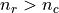reads: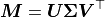where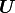is an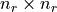orthogonal matrix,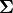is andiagonal matrix and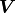is anorthogonal matrix.

Parameters:
fullSVDbool, optional

Whether the null parts of the orthogonal factors are explicitly stored or not. Default is False and computes a reduced SVD.

keep_intactbool, optional

A flag telling whether the present matrix can be overwritten or not. Default is True and leaves the present matrix unchanged.

Returns:
singular_valuesPoint

The vector of singular values with size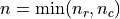that form the diagonal of thematrixof the SVD.

USquareMatrix

The left orthogonal matrix of the SVD.

VTSquareMatrix

The transposed right orthogonal matrix of the SVD.

Notes

This uses LAPACK’s DGESDD.

Examples

>>> import openturns as ot
>>> import numpy as np
>>> M = ot.Matrix([[1.0, 2.0], [3.0, 4.0], [5.0, 6.0]])
>>> singular_values, U, VT = M.computeSVD(True)
>>> Sigma = ot.Matrix(M.getNbRows(), M.getNbColumns())
>>> for i in range(singular_values.getSize()):
...     Sigma[i, i] = singular_values[i]
>>> np.testing.assert_array_almost_equal(U * Sigma * VT, M)

computeSingularValues(keepIntact=True)

Compute the singular values.

Parameters:
fullSVDbool, optional

Whether the null parts of the orthogonal factors are explicitly stored or not. Default is False and computes a reduced SVD.

keep_intactbool, optional

A flag telling whether the present matrix can be overwritten or not. Default is True and leaves the present matrix unchanged.

Returns:
singular_valuesPoint

The vector of singular values with sizethat form the diagonal of thematrixof the SVD decomposition.

Examples

>>> import openturns as ot
>>> M = ot.Matrix([[1.0, 2.0], [3.0, 4.0], [5.0, 6.0]])
>>> print(M.computeSingularValues(True))
[9.52552,0.514301]

computeSumElements()

Compute the sum of the matrix elements.

Returns:
suma float

The sum of the elements.

Notes

We compute here the sum of elements of the matrix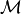, that defines as: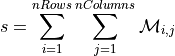Examples

>>> import openturns as ot
>>> M = ot.Matrix([[1.0, 2.0], [3.0, 4.0], [5.0, 6.0]])
>>> s = M.computeSumElements()
>>> print(s)
21.0

computeTrace()

Compute the trace of the matrix.

Returns:
tracefloat

The trace of the matrix.

Examples

>>> import openturns as ot
>>> M = ot.SquareMatrix([[1.0, 2.0], [3.0, 4.0]])
>>> M.computeTrace()
5.0

getClassName()

Accessor to the object’s name.

Returns:
class_namestr

The object class name (object.__class__.__name__).

getDiagonal(k=0)

Get the k-th diagonal of the matrix.

Parameters:
kint

The k-th diagonal to extract Default value is 0

Returns:
D: Matrix

The k-th diagonal.

Examples

>>> import openturns as ot
>>> M = ot.Matrix([[1.0, 2.0, 3.0], [4.0, 5.0, 6.0], [7.0, 8.0, 9.0]])
>>> diag = M.getDiagonal()
>>> print(diag)
[[ 1 ]
[ 5 ]
[ 9 ]]
>>> print(M.getDiagonal(1))
[[ 2 ]
[ 6 ]]

getDiagonalAsPoint(k=0)

Get the k-th diagonal of the matrix.

Parameters:
kint

The k-th diagonal to extract Default value is 0

Returns:
ptPoint

The k-th digonal.

Examples

>>> import openturns as ot
>>> M = ot.Matrix([[1.0, 2.0, 3.0], [4.0, 5.0, 6.0], [7.0, 8.0, 9.0]])
>>> pt = M.getDiagonalAsPoint()
>>> print(pt)
[1,5,9]

getDimension()

Accessor to the dimension (the number of rows).

Returns:
dimensionint
getId()

Accessor to the object’s id.

Returns:
idint

Internal unique identifier.

getImplementation()

Accessor to the underlying implementation.

Returns:
implImplementation

A copy of the underlying implementation object.

getName()

Accessor to the object’s name.

Returns:
namestr

The name of the object.

getNbColumns()

Accessor to the number of columns.

Returns:
n_columnsint
getNbRows()

Accessor to the number of rows.

Returns:
n_rowsint
isDiagonal()

Test whether the matrix is diagonal or not.

Returns:
testbool

isEmpty()

Tell if the matrix is empty.

Returns:
is_emptybool

True if the matrix contains no element.

Examples

>>> import openturns as ot
>>> M = ot.Matrix([[]])
>>> M.isEmpty()
True

isPositiveDefinite()

Test whether the matrix is positive definite or not.

A matrixis positive definite if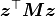is positive for every compatible non-zero column vector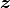.

Returns:
testbool

Notes

This uses LAPACK’s DPOTRF.

reshape(newRowDim, newColDim)

Reshape the matrix.

Parameters:
newRowDimint

The row dimension of the reshaped matrix.

newColDimint

The column dimension of the reshaped matrix.

Returns:
MTMatrix

The reshaped matrix.

Notes

If the size of the reshaped matrix is smaller than the size of the matrix to be reshaped, only the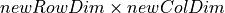first elements are kept (in a column-major storage sense). If the size is greater, the new elements are set to zero.

Examples

>>> import openturns as ot
>>> M = ot.Matrix([[1.0, 2.0], [3.0, 4.0], [5.0, 6.0]])
>>> print(M)
[[ 1 2 ]
[ 3 4 ]
[ 5 6 ]]
>>> print(M.reshape(1, 6))
1x6
[[ 1 3 5 2 4 6 ]]
>>> print(M.reshape(2, 2))
[[ 1 5 ]
[ 3 2 ]]
>>> print(M.reshape(2, 6))
2x6
[[ 1 5 4 0 0 0 ]
[ 3 2 6 0 0 0 ]]

reshapeInPlace(newRowDim, newColDim)

Reshape the matrix, in place.

Parameters:
newRowDimint

The row dimension of the reshaped matrix.

newColDimint

The column dimension of the reshaped matrix.

Notes

If the size of the reshaped matrix is smaller than the size of the matrix to be reshaped, only thefirst elements are kept (in a column-major storage sense). If the size is greater, the new elements are set to zero. If the size is unchanged, no copy of data is done.

Examples

>>> import openturns as ot
>>> M = ot.Matrix([[1.0, 2.0], [3.0, 4.0], [5.0, 6.0]])
>>> print(M)
[[ 1 2 ]
[ 3 4 ]
[ 5 6 ]]
>>> M.reshapeInPlace(1, 6)
>>> print(M)
1x6
[[ 1 3 5 2 4 6 ]]
>>> M.reshapeInPlace(2, 2)
>>> print(M)
[[ 1 5 ]
[ 3 2 ]]
>>> M.reshapeInPlace(2, 6)
>>> print(M)
2x6
[[ 1 5 0 0 0 0 ]
[ 3 2 0 0 0 0 ]]

setDiagonal(*args)

Set the k-th diagonal of the matrix.

Parameters:
diagPoint or Matrix or a float

Value(s) used to fill the diagonal of the matrix

kint

The k-th diagonal to fill Default value is 0

Examples

>>> import openturns as ot
>>> M = ot.Matrix([[1.0, 2.0, 3.0], [4.0, 5.0, 6.0], [7.0, 8.0, 9.0]])
>>> M.setDiagonal([-1, 23, 9])
>>> print(M)
[[ -1  2  3 ]
[  4 23  6 ]
[  7  8  9 ]]
>>> M.setDiagonal(1.0)
>>> print(M)
[[ 1 2 3 ]
[ 4 1 6 ]
[ 7 8 1 ]]
>>> M.setDiagonal([2, 6, 9])
>>> print(M)
[[ 2 2 3 ]
[ 4 6 6 ]
[ 7 8 9 ]]

setName(name)

Accessor to the object’s name.

Parameters:
namestr

The name of the object.

solveLinearSystem(*args)

Solve a square linear system whose the present matrix is the operator.

Parameters:
rhssequence of float or Matrix with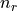values or rows, respectively

The right hand side member of the linear system.

Returns:
solution

The solution of the square linear system.

Notes

This will handle both matrices and vectors. Note that you’d better type explicitly the matrix if it has some properties that could simplify the resolution (see TriangularMatrix).

This uses LAPACK’S DGESV for matrices and DGELSY for vectors.

Examples

>>> import openturns as ot
>>> import numpy as np
>>> M = ot.SquareMatrix([[1.0, 2.0], [3.0, 4.0]])
>>> b = [1.0] * 2
>>> x = M.solveLinearSystem(b)
>>> np.testing.assert_array_almost_equal(M * x, b)

solveLinearSystemInPlace(*args)

Solve a rectangular linear system whose the present matrix is the operator.

Similar to solveLinearSystem() except the matrix is modified in-place during the resolution avoiding the need to allocate an extra copy if the original copy is not re-used.

squareElements()

Square the Matrix, ie each element of the matrix is squared.

Examples

>>> import openturns as ot
>>> M = ot.Matrix([[1.0, 2.0], [3.0, 4.0], [5.0, 6.0]])
>>> M.squareElements()
>>> print(M)
[[  1  4 ]
[  9 16 ]
[ 25 36 ]]

transpose()

Transpose the matrix.

Returns:
MTSquareMatrix

The transposed matrix.

Examples

>>> import openturns as ot
>>> M = ot.SquareMatrix([[1.0, 2.0], [3.0, 4.0]])
>>> print(M)
[[ 1 2 ]
[ 3 4 ]]
>>> print(M.transpose())
[[ 1 3 ]
[ 2 4 ]]


## Examples using the class¶Estimate Wilks and empirical quantile

Estimate Wilks and empirical quantileEstimate a multivariate distribution

Estimate a multivariate distributionTest independence

Test independenceFit a parametric copula

Fit a parametric copulaFit a non parametric copula

Fit a non parametric copulaVisualize pairs

Visualize pairsVisualize clouds

Visualize cloudsCreate and draw multivariate distributions

Create and draw multivariate distributionsQuick start guide to distributions

Quick start guide to distributionsDraw minimum volume level sets

Draw minimum volume level setsCreate a copula

Create a copulaExtract the copula from a distribution

Extract the copula from a distributionAssemble copulas

Assemble copulasCreate a functional basis process

Create a functional basis processCreate a parametric spectral density function

Create a parametric spectral density functionExport a field to VTK

Export a field to VTKCreate a stationary covariance model

Create a stationary covariance modelCreate a normal process

Create a normal processDraw a field

Draw a fieldSample trajectories from a Gaussian Process with correlated outputs

Sample trajectories from a Gaussian Process with correlated outputsDraw fields

Draw fieldsMixture of experts

Mixture of expertsKriging :configure the optimization solver

Kriging :configure the optimization solverEstimate moments from Taylor expansions

Estimate moments from Taylor expansionsUse the post-analytical importance sampling algorithm

Use the post-analytical importance sampling algorithmSubset Sampling

Subset SamplingCreate unions or intersections of events

Create unions or intersections of eventsCreate an event based on a process

Create an event based on a processEstimate Sobol indices on a field to point function

Estimate Sobol indices on a field to point functionParallel coordinates graph as sensitivity tool

Parallel coordinates graph as sensitivity toolUse the ANCOVA indices

Use the ANCOVA indicesCreate mixed deterministic and probabilistic designs of experiments

Create mixed deterministic and probabilistic designs of experimentsCalibration without observed inputs

Calibration without observed inputsIterated Functions System

Iterated Functions SystemControl algorithm termination

Control algorithm terminationA quick start guide to graphs

A quick start guide to graphs